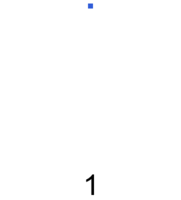# Pentagonal Number TheoremFrom the previous problems, we have learnt that the Pentagonal Numbers are given by the formula $p_n = \frac{ 3n^2- n} { 2}$. We have investigated the sequence for positive integers $n$, which gives us the values:

$1, 5, 12, 22, 35, 51, \ldots$

What happens when we substitute in non-positive integers into this formula? We will get a different sequence, namely:

$0, 2, 7, 15, 26, 40, 57, \ldots$

Together, these numbers are called the Generalized Pentagonal Numbers. They appear in the following theorem:

The Pentagonal Number Theorem states that
$\prod_{n=1}^\infty ( 1 - x^n) = \sum_{k=-\infty}^{\infty} (-1)^k x^{p_k} = 1 - x - x^2 + x^5 + x^7 - x^{12} - x^{15} \ldots$

If we take the combinatorial interpretation of the identity, the LHS is the generating function for the number of partitions of $n$ into an even number of distinct parts, minus the number of partitions of $n$ into an odd number of distinct parts. By looking at the coefficients on the RHS, the surprising corollary is that this difference is either -1, 0 or 1 always!

The combinatorial proof of the Pentagonal Number Theorem follows a similar argument. It creates a bijection between the number of even parts, and the number of odd parts, and then accounts for the cases where the bijection fails, namely at the values $p_k = \frac{ 3k^2 - k } { 2 }$. You can attempt to prove it for yourself, or read the Wikipedia article.Note by Calvin Lin
7 years, 2 months ago

This discussion board is a place to discuss our Daily Challenges and the math and science related to those challenges. Explanations are more than just a solution — they should explain the steps and thinking strategies that you used to obtain the solution. Comments should further the discussion of math and science.

When posting on Brilliant:

• Use the emojis to react to an explanation, whether you're congratulating a job well done , or just really confused .
• Ask specific questions about the challenge or the steps in somebody's explanation. Well-posed questions can add a lot to the discussion, but posting "I don't understand!" doesn't help anyone.
• Try to contribute something new to the discussion, whether it is an extension, generalization or other idea related to the challenge.

MarkdownAppears as
*italics* or _italics_ italics
**bold** or __bold__ bold
- bulleted- list
• bulleted
• list
1. numbered2. list
1. numbered
2. list
Note: you must add a full line of space before and after lists for them to show up correctly
paragraph 1paragraph 2

paragraph 1

paragraph 2

[example link](https://brilliant.org)example link
> This is a quote
This is a quote
    # I indented these lines
# 4 spaces, and now they show
# up as a code block.

print "hello world"
# I indented these lines
# 4 spaces, and now they show
# up as a code block.

print "hello world"
MathAppears as
Remember to wrap math in $$ ... $$ or $ ... $ to ensure proper formatting.
2 \times 3 $2 \times 3$
2^{34} $2^{34}$
a_{i-1} $a_{i-1}$
\frac{2}{3} $\frac{2}{3}$
\sqrt{2} $\sqrt{2}$
\sum_{i=1}^3 $\sum_{i=1}^3$
\sin \theta $\sin \theta$
\boxed{123} $\boxed{123}$

Sort by:

nice

- 7 years, 2 months ago

Does it work for n-gon as well? I haven't tried to generalize it yet........

- 7 years, 2 months ago

Does what work for n-gon?

If you're referring to finding the formula for the number of dots in Figure K of an N-gon diagram, then yes you can use similar approaches. The easiest will be to find the Arithmetic Progression, and then find the sum of that. I wanted to show a different approach, which uses the idea of Mathematical Induction, or Method of Differences.

Staff - 7 years, 2 months ago

I meant the number of dots only. Thanks, sir.

- 7 years, 2 months ago

Why don't you investigate and then write up a note? I'd be interested in your results.

Staff - 7 years, 2 months ago

great

- 7 years, 2 months ago

Greaat :) It was very interesting. Thank you !

- 7 years, 2 months ago

it would be always positive integer.

- 7 years, 2 months ago

i will continue my thinking unless i will got it. Thanks

- 7 years, 1 month ago

Fantastic

- 7 years ago

Nice one sir! Can I suggest something? Knowing 1st pentagonal number is one, we can see that number of dots to be added to form the next pentagonal number are in A.P. - where a=4, and common difference d=3.... Thus can't we express pentagonal numbers as sums of the increasing A.P. 1,4,7,10; i.e. S1, S2, S3 and so on, where S(n) is the sum to 'n' terms of the A.P. 1,4,7,10...?

- 6 years, 9 months ago